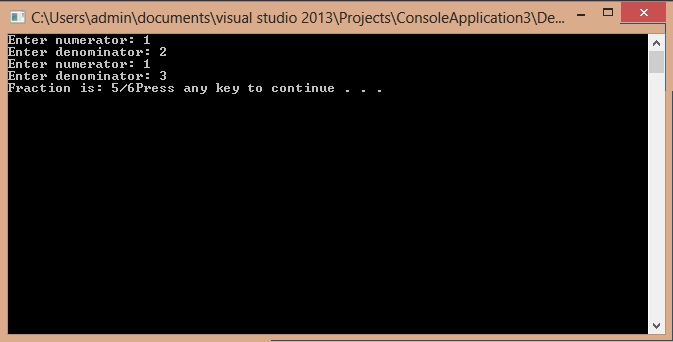`#include<iostream>#include<conio.h>using namespace std;`
`class fraction{private:			//access specifier 				int num;	int denom;public:	void getfraction();	fraction operator + (fraction f);	friend istream& operator >> (istream &input, fraction &f); }; void fraction::getfraction(){	cout << "Fraction is: " << num << "/" << denom << endl;}  istream& operator >> (istream &input, fraction &f){	cout << "Enter the numerator: ";	input >> f.num;	cout << "Enter the denominator: ";	input >> f.denom; 	return input;} fraction fraction :: operator + (fraction f){	fraction sum;	sum.num = (this->num*f.denom) + (this->denom*f.num);	sum.denom = this->denom*f.denom; 	return sum;}`
`int main(){	fraction f1;		//initializing a variable f1 of type fraction	fraction f2;		//initializing a variable f2 of type fraction	cin >> f1;	cin >> f2; 	fraction f3; 	f3 = f1 + f2; 	f3.getfraction(); 	system("pause");}`
In the main function we are getting the values of f1 and f2 from the user using the overloaded operator of console input. Then we are performing the addition of these two fractions by again using an overloaded operator of +. Their sum is being stored in f3 which is later printed on the screen. Output